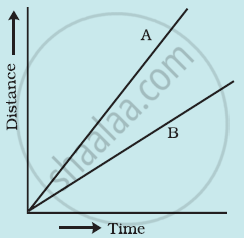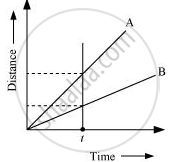Share

# Figure Shows the Distance-time Graph for the Motion of Two Vehicles a and B. Which One of Them is Moving Faster? - Science

Course

#### Question

Figure shows the distance-time graph for the motion of two vehicles A and B. Which one of them is moving faster?Figure: Distance-time graph for the motion of two cars

#### Solution

Vehicle A is moving faster than vehicle B.Speed is given by the relation

Speed = "Distance covered"/"Time taken"

This relation shows that speed of a vehicle is greater if it covers maximum distance in a given interval of time. To compare the distance, draw a line perpendicular to the time-axis, as shown in the following distance-time graphFrom the graph, it is evident that for a given time t, the distance covered by vehicle A is more than vehicle B. Hence, vehicle A is moving faster than vehicle B.

Is there an error in this question or solution?